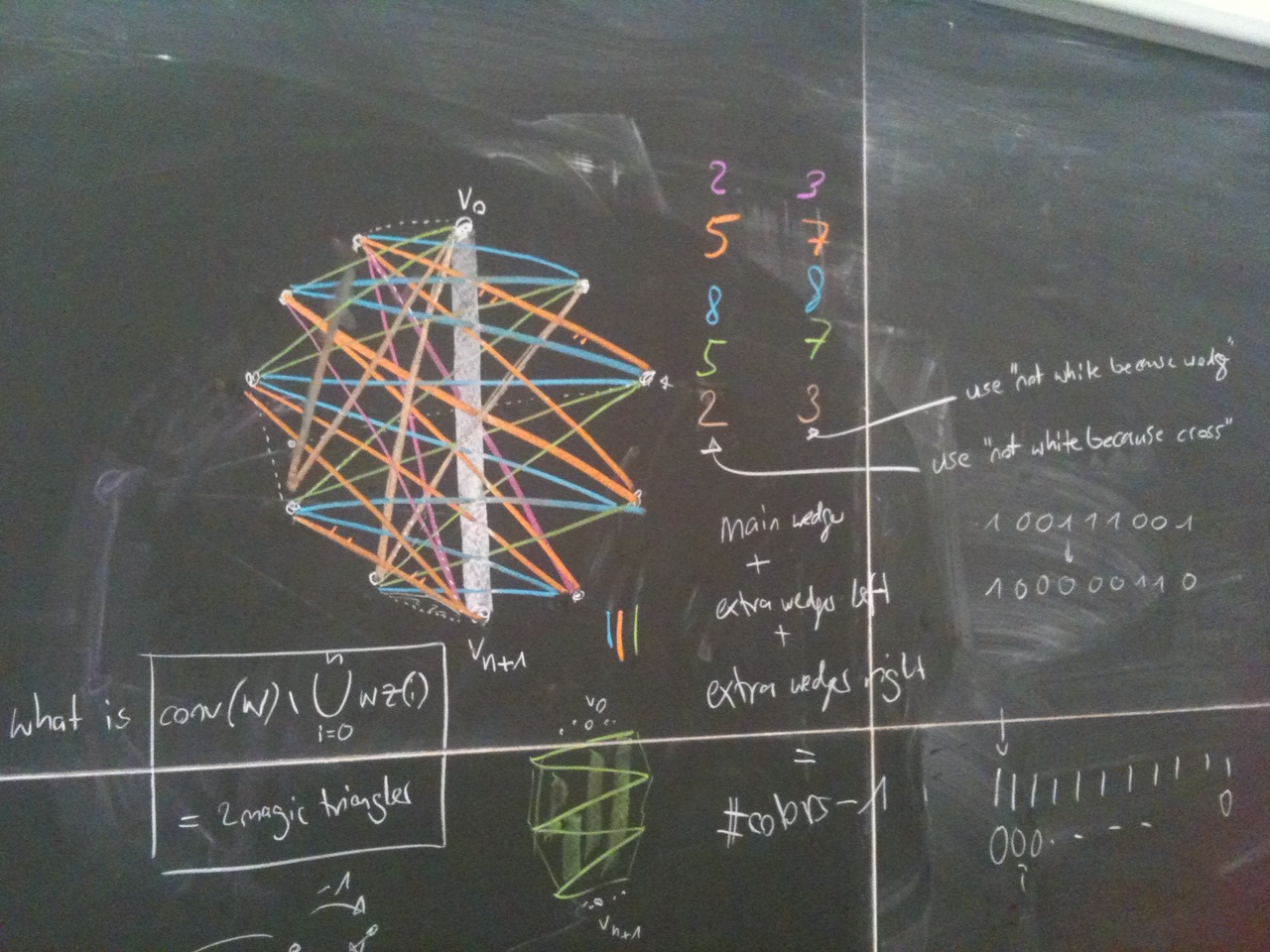## Home / Research

### My Research

For a list of my publications, visit my profiles on Google Scholar and dblp.

Topological data analysis is a relatively young subfield of data science which uses ideas and methods from algebraic topology to study the shape of data. Despite only having been introduced a bit more than 20 years ago, topological data analysis has already found many applications. From a mathematicians perspective, I also appreciate the beautiful mathematics that lie at the core of the theory. In my research I am particularly interested in the stability and robustness of persistent homology and the Mapper algorithm, and in my work I try to apply concepts from discrete geometry to the pipeline of topological data analysis. For more background, I have also written some lecture notes.
Depth measures are a way to generalize the concept of a median to higher dimensions. Several different depth measures have been introduced in the literature, all of which lead to interesting combinatorial and computational questions. I am particularly interested in the relationship between different measures and the depth structures that they induce on a data set in high dimensions.
The well-known Ham-Sandwich Theorem states that any d masses in d-dimensional space can be simultaneously bisected by a single hyperplane. The proof of this result uses tools from algebraic topology, namely the famous Borsuk-Ulam Theorem. A natural question now is to ask, whether we can bisect even more masses, if we use more or different types of cuts. For many such questions, new topological tools, similar to the Borsuk-Ulam Theorem, are required.### Some selected papers

Here you find a selection of some of my recent papers.

#### Enclosing Depth and other Depth MeasuresCombinatorica 2023

I study families of depth measures defined by natural sets of axioms. I show that any such depth measure is a constant factor approximation of Tukey depth. I further investigate the dimensions of depth regions, showing that the Cascade conjecture, introduced by Kalai for Tverberg depth, holds for all depth measures which satisfy the most restrictive set of axioms, which includes Tukey depth. Along the way, I introduce and study a new depth measure called enclosing depth, which I believe to be of independent interest, and show its relation to a constant-fraction Radon theorem on certain two-colored point sets.

#### Combinatorial Depth Measures for Hyperplane ArrangementsSoCG 2023

Regression depth, introduced by Rousseeuw and Hubert in 1999, is a notion that measures how good of a regression hyperplane a given query hyperplane is with respect to a set of data points. Under projective duality, this can be interpreted as a depth measure for query points with respect to an arrangement of data hyperplanes. The study of depth measures for query points with respect to a set of data points has a long history, and many such depth measures have natural counterparts in the setting of hyperplane arrangements. For example, regression depth is the counterpart of Tukey depth. Motivated by this, together with Pablo Soberon we study general families of depth measures for hyperplane arrangements and show that all of them must have a deep point. Along the way we prove a Tverberg-type theorem for hyperplane arrangements, giving a positive answer to a conjecture by Rousseeuw and Hubert from 1999. We also get three new proofs of the centerpoint theorem for regression depth, all of which are either stronger or more general than the original proof by Amenta, Bern, Eppstein, and Teng. Finally, we prove a version of the center transversal theorem for regression depth.

#### A Topological Version of Schaefer's Dichotomy TheoremarXiv 2023

Schaefer's dichotomy theorem states that a boolean constraint satisfaction problem (CSP) is polynomial-time solvable if one of six given conditions holds for every type of constraint allowed in its instances. Otherwise, it is NP-complete. In this paper, together with Simon Weber we analyze boolean CSPs in terms of their topological complexity, instead of their computational complexity. We attach a natural topological space to the set of solutions of a boolean CSP and introduce the notion of projection-universality. We prove that a boolean CSP is projection-universal if and only if it is categorized as NP-complete by Schaefer's dichotomy theorem, showing that the dichotomy translates exactly from computational to topological complexity. We show a similar dichotomy for SAT variants and homotopy-universality.